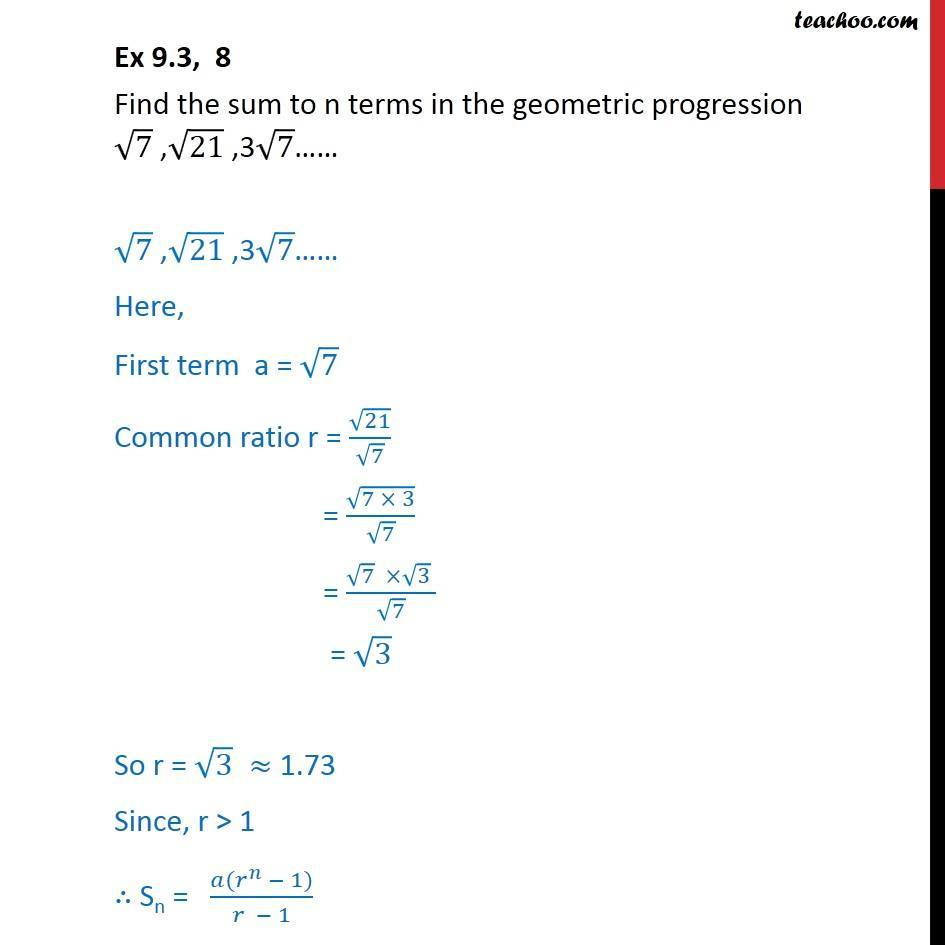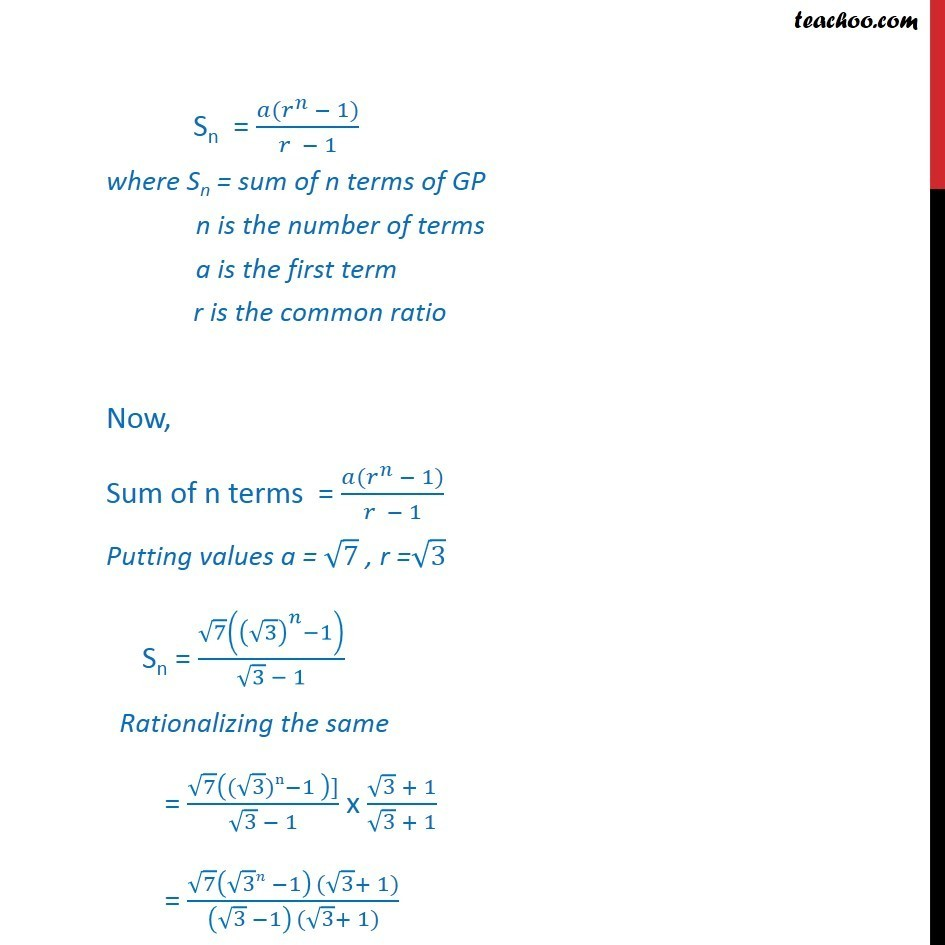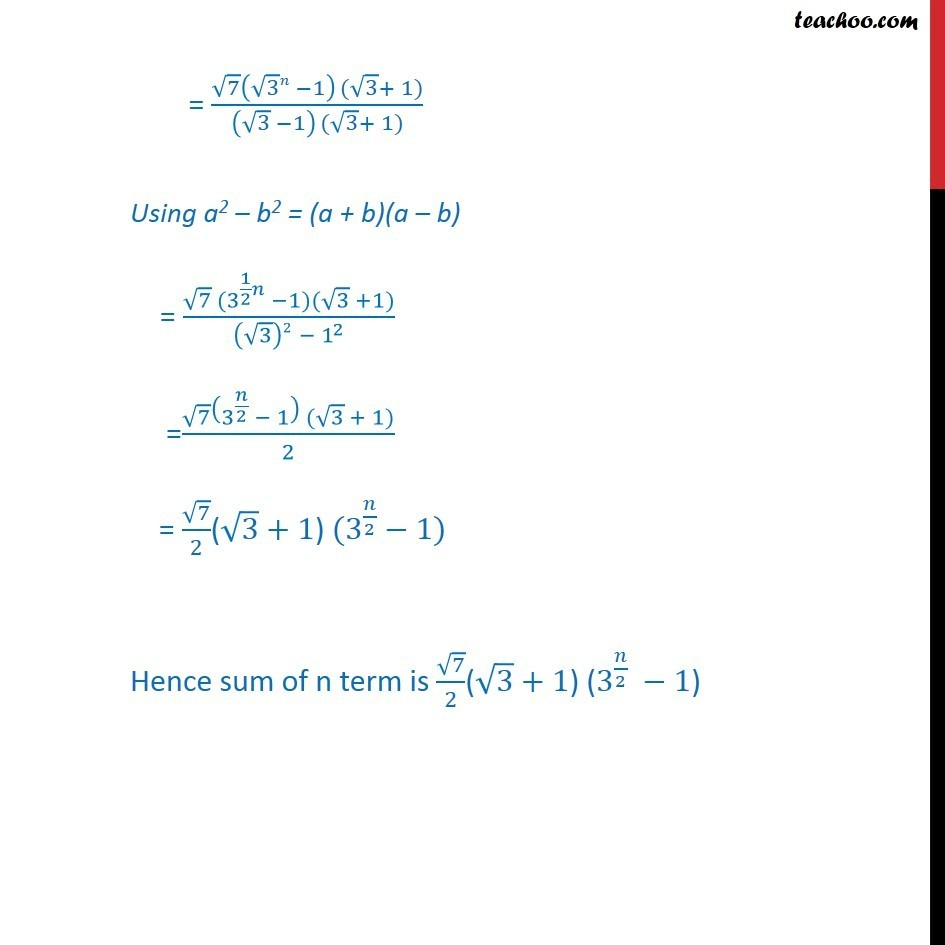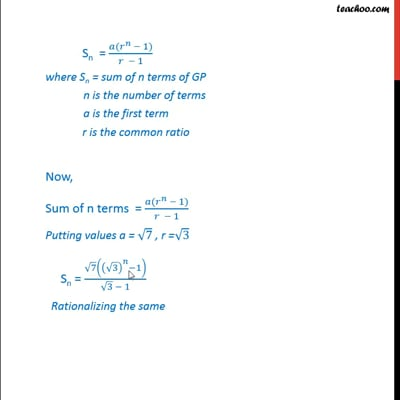Ex 9.3

Chapter 9 Class 11 Sequences and Series
Serial order wiseThis video is only available for Teachoo black users

Solve all your doubts with Teachoo Black (new monthly pack available now!)

### Transcript

Ex 9.3, 8 Find the sum to n terms in the geometric progression 7 , 21 ,3 7 7 , 21 ,3 7 Here, First term a = 7 Common ratio r = 21/ 7 = (7 3)/ 7 = ( 7 3 )/ 7 = 3 So r = 3 1.73 Since, r > 1 Sn = ( ( ^ 1))/( 1) Sn = ( ( ^ 1))/( 1) where Sn = sum of n terms of GP n is the number of terms a is the first term r is the common ratio Now, Sum of n terms = ( ( ^ 1))/( 1) Putting values a = 7 , r = 3 Sn = ( 7 (( 3)^ 1))/( 3 1) Rationalizing the same = ( 7 (( 3)n 1 )])/( 3 1) x ( 3 + 1)/( 3 + 1) = ( 7 ( 3 1) ( 3+ 1))/(( 3 1) ( 3+ 1)) = ( 7 ( 3 1) ( 3+ 1))/(( 3 1) ( 3+ 1)) Using a2 b2 = (a + b)(a b) = ( 7 (3^(1/2 ) 1)( 3 +1))/(( 3)2 1^2 ) =( 7 (3^( /2) 1) ( 3 + 1))/2 = 7/2( 3+1) (3^( /2) 1) Hence sum of n term is 7/2( 3+1) (3^( /2) 1)